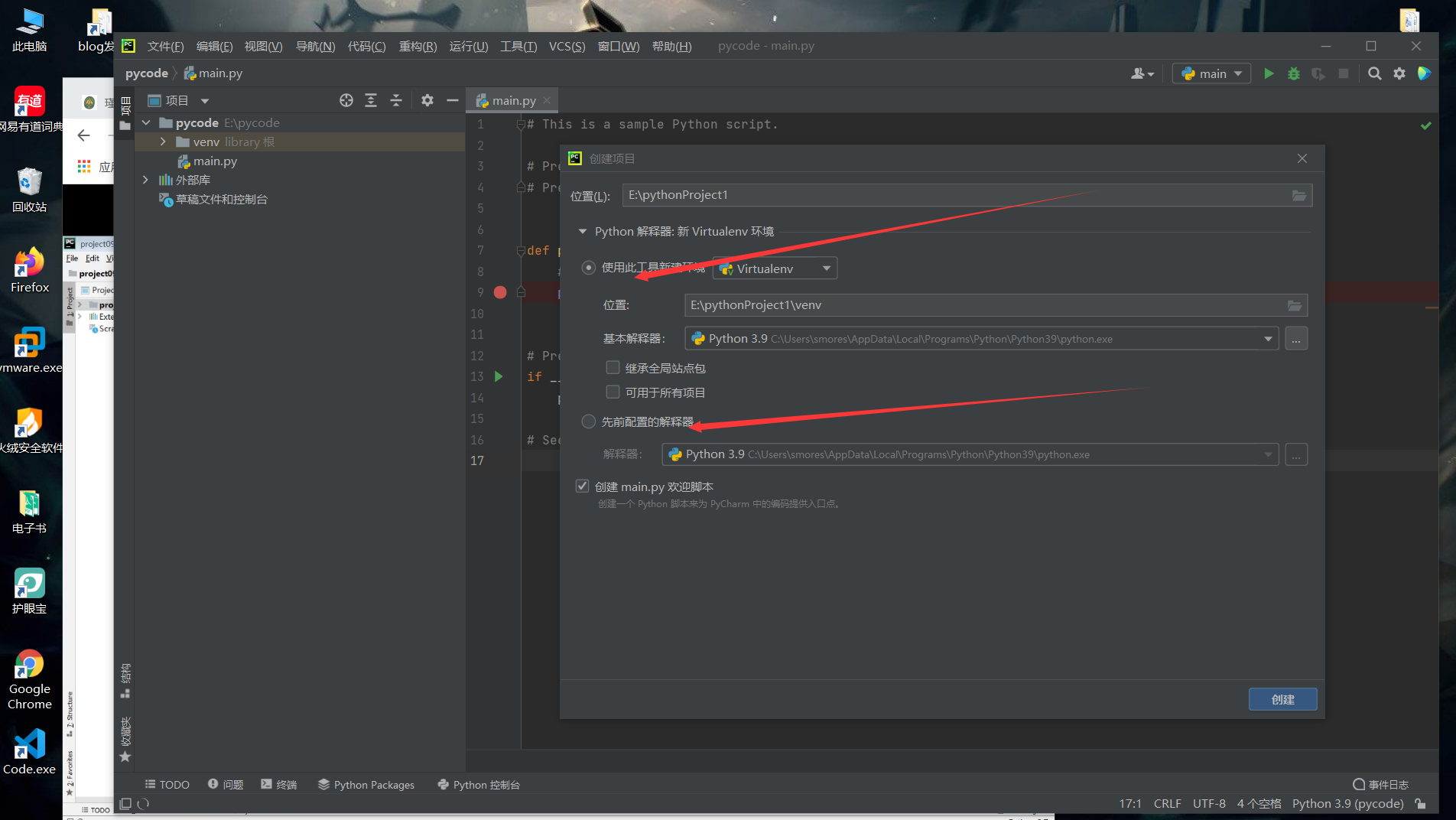# python的一些入门基础知识

## p1-Python的介绍

``````#输入成绩判断等级：

cj=float (input("请输入你的成绩："))
if cj>=90 and cj<=100:
print("优秀")
elif cj>=70 and cj<90:
print("良好")
elif cj>=60 and cj<70:
print("及格")
else:
print("你要努力了")``````

## p2-环境pycharm等安装

### p2-Python安装

https://mp.weixin.qq.com/s/4oWzRIn0sasfrxwpli_4hw

pip使用教程（下载标准库外的库）

https://www.cnblogs.com/songzhixue/p/11296720.html

### Pycharm安装

``https://mp.weixin.qq.com/s?__biz=MzI3NzI1MzY4Mw==&mid=2247492904&idx=1&sn=0970650063d724b9c251848a2e5789ad&chksm=eb6bbe74dc1c3762518dbeb3bc2813ca8da339bfc014bf897fc2450d431f5a29343ceccfbbe4&scene=178&cur_album_id=1786174076420472834#rd``

cd命令

``````https://jingyan.baidu.com/article/6079ad0ec0145b28ff86db88.html

###开发环境介绍

venv与直接建立项目的区别

venv建立项目是创造一个新的环境，库与标准库等是分开的，如当你接私活爬虫的requests库需要用2.0版本，而公司项目用的是1.0版本，如果不用venv虚拟隔开，就会冲突。### Pycharm

``````https://blog.csdn.net/cuomer/article/details/81534140

https://www.cnblogs.com/sui776265233/p/10200809.html

crtl+/注释
ctrl+alt+l格式化代码
ctrl+d复制当前行到下一行
ctrl+y删除当前行

``````

win+r打开运行菜单，snippingtool系统截图工具

mspaint是画图工具

## p3-基础

### 小知识

``````a = 1
b = 2
print(a,b,100)
#结果为1 2 100，print可以多个输出，而且可以直接带已知数,下面是print函数定义
print("-"*20)
#结果为二十个"-"号--------------------，别问为什么，就是这样设置的
#如果是数字和字符串做乘法运算，会将这个字符串重复多次。
print()中整形也不能与字符串拼接，字符串双引号和单引号都可以。
end="/n"即语句输出完最后加一个换行，sep=":"即每个输出间用":"隔开

​```def print(self, *args, sep=' ', end='\n', file=None): # known special case of print
"""
print(value, ..., sep=' ', end='\n', file=sys.stdout, flush=False)

Prints the values to a stream, or to sys.stdout by default.
Optional keyword arguments:
file:  a file-like object (stream); defaults to the current sys.stdout.
sep:   string inserted between values, default a space.
end:   string appended after the last value, default a newline.
flush: whether to forcibly flush the stream.
"""
pass
```

``````

### 变量命名

python中直接a = 100，A = ‘ABC’即可，不需要int和string什么的，会自动帮我们定义。

``````money = 200
type(money)#看money的类型
print(type(money))#现在是int
money = 20.2
print(type(money))#现在是float
money = '1万'
print(type(money))#现在是str字符串，在引号里面就是字符串，单引号双引号都可以。``````

1-分大小写
2-由字母，数字，下划线，其他的特殊符号不行，不能数字开头。
3-格式错误可运行，有灰色波浪线，可用中文，但会黄色警告。
4-不能使用关键字。
5-最好见名知意
6-小驼峰开头第一个字母全部小写如getNameByLine,但python最好用下划线，如get_name_by_line
7-大驼峰，每个字母大写GetNameByLine（在面向对象的类名中使用）

### 数据类型与转换``````message = "'abcdefg'"
message = '"abcdefg"'

### 类型转换``````输入money等于10

``````input输入的默认是字符串类型，赋值后变量是字符串。

int型可随便转成str型### 赋值与输出

``````a = b = c = 5#可
d="dddd"
a,b,c,d=5,5,5,"ddd"#可
print(a,b,c,d)
print("%daaaaaaaa      %d%d%s" %(a,b,c,d))#这时输出后a和b相差aaaaaa和多个空格，print里面怎么写就输出什么``````

### 算术运算符### 关系运算符### 逻辑运算符

and两边一边为0,结果就为0,即一侧为假就是假,如过无0,则取后面那个(and第一个判断处理后，还需要判断后一个条件，最后判断是谁就输出谁),如a=1 b=3 print(b and a),得到的是1

or两边一个为真就是真

``````a = 1
b = 2
print(a or b)
#结果为1，因为第一个1为真，就不再运行判断后面b是真是假，直接输出1
a = 0
b = 2
print(a or b)
#结果为2，因为第一个是0假，再判断后面的，后面为2，就输出2
#这里是输出数字，在编辑器中已经定义了就是这样运行的``````
``````a = 1
b = 2
c = 3
print(b > a and b<3)
#结果为true，这里不是数字，而有关系运算符，就输出true或者false
print(not (b > a and b<3))
#结果为false，not逻辑就是取反
``````

### 格式化输出%d digit数字数位，%s string ,%f float ,%o octal八进制

``````age = 20
name = "陈冠希"
money = 2000000.99
print('%d岁的%s一部电影赚了%.2f元' % (age,name,money))
#注意print里面有''
print(age+"岁的"+name+"一部电影赚了"+money+"元")
#或者print(str(age)+'岁的'+name+'一部电影赚了'+str(money)+'元')
#直接字符串拼接，但是因为age和money不是字符串类型，无法拼接，所以必须先强转。（拼接必须全是字符串类型）

``````

%.2f即保留两位小数输出### 进制转换1，2，4，8，16，32，64，128，256 ，二进制哪一位有相加即可快速得到十进制。

``````a=bin(20)
#转二进制
print(a)
a=oct(50)
#转八进制
print(a)
a=hex(150)
#转十六进制
print(a)
#binary二进制，octal八进制，hex十六进制``````## 位运算结果1字节=1byte=8bit=三十二位### 运算符优先级### 条件语句

``````import random  # 引入随机数包

ran = random.randint(1, 10)  # random.randint(1，10)生成1到10的整数
ran2 = random.randint(1, 10)
print(ran, ran2)
abs(ran)  # abs()是将括号里面的数字绝对值
if ran > ran2:
print("yes")
else:
print("no")
``````

if-elif-else

``````#当所有的elif都不成立，才运行else，一个成立则跳出此判断语句再向下运行
sales = int(input("请输入你的销售金额，不同金额有不同奖励"))#注意int转换下类型，input是字符串类型，无法与整形比较
if 10000 < sales <=50000:
print("你得到了一辆tesla")
elif 50000 < sales <=100000:
print("你得到了两辆tesla")
elif 150000 < sales <=200000:
print("你得到了三辆tesla")
else:
print("你被开除了")``````

pass关键词

``````a = 1
if a==1 :
pass
else :
pass
#pass无意义，但可支撑这个语句，不填语句会报错，pass可当空占用``````

``````a = 10
b = 30
if a > b:
c = a
else:
c = b
print('a和b两个数的较大值是%d'%c)

if a > b:
print("a>b")
else:
print("a<b")``````

``````a = 10
b = 30
c = a if a>b else b
print('a和b两个数的较大值是%d'%c)

print("a>b") if a>b else print("a<b")``````

### 循环语句

continue和break要在for,while等循环中使用,单独的if语句中不能使用break和continue.

continue结束当前循环，break跳出整个循环

``````# i = 0
# next1 = 0
# num = 0
#
# while i < 100:
#     next1 += 1
#     i += 1
#     num = num +next1
# print(num)

# 猜数字
# 因为不知道要猜多少次所以用死循环，然后判断加break，猜对就break跳出
# import random
#
# while True:
#     ran = random.randint(1, 10)
#     guess = int(input("欢迎来到随机猜数字游戏(1-10)，请输入您猜的数字"))
#     # 记得把input转int不然永远是错
#     if guess == ran:
#         print(" 恭喜你猜对了")
#         break
#     else:
#         print("你猜错了")

# for循环
# range(10),输出0-9，默认从0开始，sep为1，不包括最后一个
# 输出0 1 2 3 4 5 6 7 8 9
# for i in range(10):
#     print(i)

# 从1开始，间隔为2，输出1 3 5 7 9
for i in range(1, 10, 2):
print(i)
``````

### for- else 和 while -else

``````for i in range(3):
if i > 3:
break
else:
print("if一直不成立，没执行过break，执行此else语句")``````

### continue和break

``````# 输出能不能被3整除的1-10的数
# for i in range(10):
#     if i % 3 != 0:
#         print(i)
#     else:
#         pass

# continue
for i in range(10):
if i % 3 == 0:
continue
else:
print(i)

# break跳出整个循环，注意是最近的循环，不是跳出if，continue是跳出当前的一次循环
``````

### 循环嵌套

``````# 打出倒立直角三角形
*
**
***
****
*****
# 方法一
# n = 0
# while n <= 5:
#     print("*" * n)
#     n += 1

# 方法二
n = 1
while n <= 5:
m = 0
while m < n:
print("*", end="")#取消换行，因为这里的*需要连起来
m += 1
print()#加换行
n += 1

``````

### 切片操作，s3=”hello”编辑器从变量池找hello，发现已经有了，就直接赋予，这么做是为了节省空间，所以s1和s2

``````#查看地址的函数 id()
n = 3
print(id(n))

#转绝对值的函数 abs()
n = -3
print(abs(n))

#查看类型type()
n = -3
print(type(n))

#获取ascll码ord()
n = "A"
print(ord(n))
#结果65

#获取字符串长度len()
n = "111"
print(len(n))
#结果3``````

### 字符串的截取## 注意事项

1-python不用{}包含语句，而是用缩进规划范围，所以在if等语句中一定要注意缩进。

2-python里没有i++这种自增

```

目录